# PSEB 7th Class Maths Solutions Chapter 1 Integers Ex 1.2

Punjab State Board PSEB 7th Class Maths Book Solutions Chapter 1 Integers Ex 1.2 Textbook Exercise Questions and Answers.

## PSEB Solutions for Class 7 Maths Chapter 1 Integers Ex 1.2

1. Find the value of:
(a) 32 + 15
(b) 17 + (-18)
(c) (-25) + (21)
(d) (-8) + (-11)
(e) (-13) + (21)
(f) (-19) + (0)
(g) (-85) – (-10)
(h) (15) – (6)
(i) (45) – (-27)
(j) (-62) – (52)
(a) 32 + 15 = 47
(b) 17 + (-18) = – 1
(c) (-25) + (21) = -4
(d) (-8) + (- 11) = – 19
(e) (-13) + (21) = 8
(f) (-19) + (0) = – 19
(g) (- 85) – (- 10) = – 85 + 10 = – 75
(h) (15) – (6) = 9
(i) (45) – (- 27) = 45 + 27 = 72
(j) (- 62) – (52) = – 62 – 52 = – 114

2. Solve the following :

Question (a).
(-3) + 7 + (-8)
(- 3) + 7 + (- 8) = (- 3) + (- 8) + 7
= – 11 + 7
[∵ (- 3) + (- 8) = – 11] = – 4

Question (b).
(- 2) – (- 1) – (4)
(- 2) – (- 1) – (4)= – 2 + 1 – 4
= (- 2) + (- 4) + 1
= – 6 + 1 = – 5Question (c).
8 + (- 7) – (- 6)
8 + (- 7) – (- 6) = 8 + (- 7) + (6)
= (8) + (6) + (- 7)
= 14 + (- 7) = 7

Question (d).
(- 12) – (- 17)+ (- 25)
(- 12) – (- 17) + (- 25) = (- 12) + (+ 17) + (- 25)
= (- 12) + (- 25) + (+ 17)
[∵ (- 12) + (- 25) = (- 37)]
= (- 37) + (+ 17)
= – 20

3. Find the value of:

Question (a).
15 – (- 5) + 12 + (- 8) + (- 3)
15 – (- 5) + 12 + (- 8) + (- 3)
= 15 + (+ 5) + 12 + (- 8) + (- 3)
= 32 + (- 11)
= 21
[∵ 15 + (+ 5) + 12 = 32 and (- 8) + (- 3) = (- 11)]

Question (b).
(- 32) – (-11) + (- 25) + 27 – 13 + (- 7)
(- 32) – (- 11) + (- 25) + 27 – 13 + (- 7)
= (- 32) + (+ 11) + (- 25) + 27 – 13 + (- 7)
= 11 + 27 + (- 32) + (- 25) – 13 + (- 7)
= 38 + (- 77)
= – 39.
[∵ 11 + 27 = 38 and (- 32) + (- 25) – 13 + (- 7) = – 77]

Question (c).
160 + (- 150) + (- 130) – (-100)
160 + (- 150) + (- 130) – (- 100)
= 160 + (- 150) + (- 130) + (+ 100)
= 160 + (+ 100) + (- 150) + (- 130)
= 260 + (- 280)
= – 20
[∵ 160 + (+ 100) = 260 and (-150) + (- 130) = – 280)]

Question (d).
25 – (- 15) + (- 12) + 21 – 65 – (- 38)
25 – (- 15) + (- 12) + 21 – 65 – (- 38)
= 25 + (+ 15) + (- 12) + 21 – 65 + (+ 38)
= 25 + (+ 15) + 21 + (+ 38) + (- 12) – 65
= 99 + (- 77)
= 22
[∵ 25 + (+ 15) + 21 + (+ 38) = 99 and (- 12) – 65 = – 77]4. Fill in the blanks using properties of addition and subtraction of integers :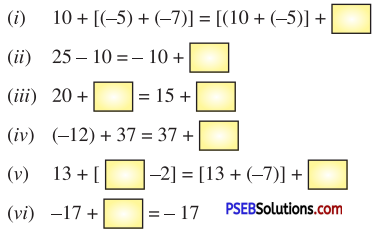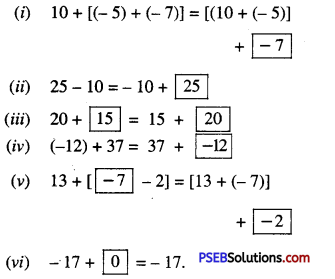5. The difference between two integers is – 10. If first integers is 17, then find the other integer ?
Difference = – 10
1st Integer = 17
2nd Integer = 1st integer – Difference
= 17 – (- 10)
= 17 + 10 = 27

6. Write three consecutive odd integers succeeding (- 93).
Three consecutive odd integers succeeding (- 93) are – 91, – 89, – 87.7. At sunrise, the outside temperature was 7° below zero. In the afternoon the temperature rose by 13° and then fell by 8° at night. What was the temperature at the end of the day ?
At sunrise, the outside temperature = 0° – 7° = -7°
In the afternoon the temperature = – 7° + 13°
= 6°
At night the temperature
= 6° – 8°
= – 2°
At the end of the day temperature – 2°.

8. Manjeet Singh has a bank balance of -₹430 at the start of the month. What was the bank balance, after he deposited ₹ 250 ?
Manjeet Singh’s bank balance in the start of the month = -₹430
Amount deposited in the bank = ₹ 250
The bank balance after depositing = -₹430 + ₹ 250
= -₹(430 + 250)
= -₹180

9. Mount Everest, the highest elevation in Asia, is 29028 feet above the sea level. The Dead Sea is 1312 feet below the sea level. What is the difference between these two elevations ?
The elevation of Mount Everest = + 29028 feet
The elevation of the Dead sea = – 1312 feet
Difference between these two elevations = [+ 29028 – (- 1312) feet
= (29098 + 1312) feet
= 30340 feet.10. In a quiz, Team A scored 70, – 15, 30. Team B scored – 15, 70, 30 and team C score 30, 70, – 15. Which team scored better ? What conclusion do you draw ?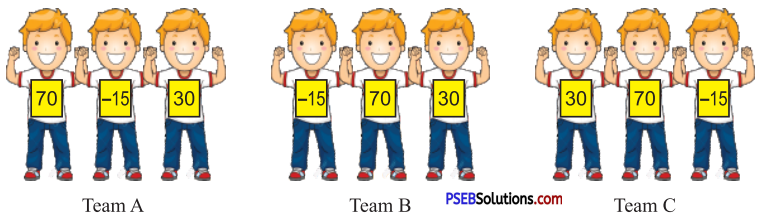Total scores scored by team A = 70 + (- 15) + 30
= 70 + 30 + (- 15)
= 100 – 15 = 85
Total scores scored by team B
= (- 15) + 70 + 30
= (- 15) + 100 = 85
Total scores scored by team C
= 30 + 70 + (- 15)
= 100 + (- 15) = 85
Scores are equal addition of integers is associative Ans.

11. In a competition there are 5 Teams and three rounds. The scores of all the teams are given below in the table. Complete the table and find, the teams at 1st, Ind and IIIrd positions.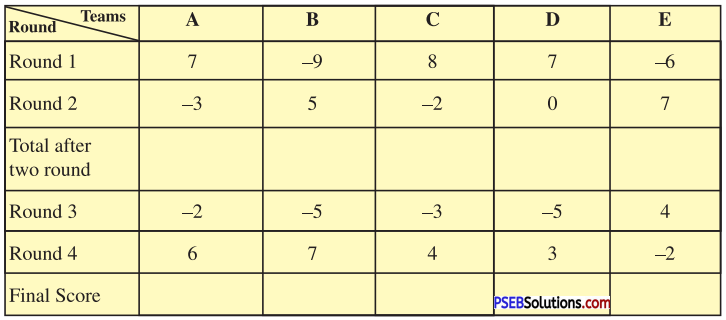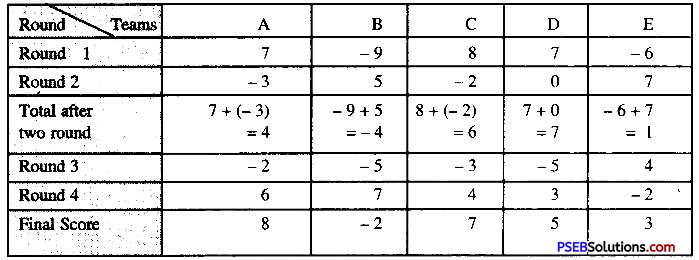Ist – A, IInd – C, III – D.

12. Multiple choice questions :

Question (i).
(- 5) + (5) =
(a) -10
(b) 5
(c) 10
(d) 0.
(d) 0.

Question (ii).
(- 10) + (- 12) =
(a) -2
(b) 22
(c) -22
(d) 2.
(c) -22Question (iii).
(- 1) – (-1) =
(a) – 2
(b) -1
(c) 2
(d) None of these.
(d) None of these.

Question (iv).
Which of the following statements is incorrect ?
(a) Sum of two integers is also an integer.
(b) For all integers a and b, a + b = b + a.
(c) Difference of two integers is also an integer.
(d) Subtraction of integers is commutative.